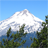Choose Language Hide Translation Bar

## Applying color schemes to data table columns

Hi - I'm trying to set up a color scheme to highlight outliers in one column of a data table, so that any value that lies outside a specified range is highlighted.  I've been using the examples for coloring cells in the Scripting Guide to see what's possible, but I can't quite get what I want.  This is what I've tried so far:

dt = open("\$SAMPLE_DATA/Big Class.jmp");

// I can color specific cells of the :name column;

:name << color cells(yellow, {1, 5, 8});

// I can color a range of the :height column with a gradient;

:height << set property("Color Gradient", {"White to Green", range(50, 80)});

:height << color cell by value(1);

// I can color 12=light red, 13=light yellow, 14=light green and 15=light blue (I don't specify 16 or 17);

:age << set property("Value Colors", {12={1,0.8,0.8}, 13={1,1,.8}, 14={.8,1,.8}, 15={.8,.8,1}});

:age << color cell by value(1);

Can anyone see a simple modification of what I've been trying to do above that would, for example, paint every value in the Weight column outside the range 80-100 red?  Many thanks.

1 ACCEPTED SOLUTION

Accepted Solutions

## Re: Applying color schemes to data table columns

You can use <<get rows where(condition) to obtain a matrix of rows that meets the specified condition.

This should work for your example:

dt = Open( "\$SAMPLE_DATA/Big Class.jmp" );

:weight << color cells( "red", dt << get rows where( :weight > 100 | :weight < 80 ) );

6 REPLIES 6

## Re: Applying color schemes to data table columns

You can use <<get rows where(condition) to obtain a matrix of rows that meets the specified condition.

This should work for your example:

dt = Open( "\$SAMPLE_DATA/Big Class.jmp" );

:weight << color cells( "red", dt << get rows where( :weight > 100 | :weight < 80 ) );

## Re: Applying color schemes to data table columns

That's exactly what I needed - many thanks - and I've just discovered that I can clear them all again by repeating the command using "black" instead of "red".  On a related point, do you know if there is there a way I can make this dynamic, so that the color potentially changes if I enter a new value into one of the cells?  I suppose what I'm effectively asking is whether I can define a property that that will automatically run a script each time I enter a value into a cell.

## Re: Applying color schemes to data table columns

Unfortunately the property Value Colors only applies to nominal or ordinal data. I am not able to set the Value Color property manually to a continuous column.

But below is "hack" that seems to work, but I am not sure how stable it is (JMP crashed with a slightly different formula). It utilizes a hidden formula column that sets the color of the weight cells according to the chosen criterion. When values are changed or new rows added the color changes accordingly. You may see a silent error message in the log, probably related to forcing the value colors on continuous column, but the formula evaluation seems to disregard this.

dt = Open( "\$SAMPLE_DATA/Big Class.jmp" );

dt << New Column( "weight color",

Formula(

:weight << color cells( "red", Data Table( "Big Class.jmp" ) << get rows where( :weight > 100 | :weight < 80 ) )

<< color cells( "white", Data Table( "Big Class.jmp" ) << get rows where( 80 <= :weight <= 100 ) )

),

hide

);

## Re: Applying color schemes to data table columns

I've just tried a few variants of the above and crashed it a few times myself - but never mind: it's useful to know that there's probably not a simple way of doing this.  For the time being I'll therefore just add a short "Update Now" script to the table which clears all the colors in the column and then reapplies them.

Many thanks again!connect01
Staff

## Re: Applying color schemes to data table columns

The silent error message in the log comes from the fact that result of the expression

:weight << color cells( "red", Data Table( "Big Class.jmp" ) << get rows where( :weight > 100 | :weight < 80 ) )

<< color cells( "white", Data Table( "Big Class.jmp" ) << get rows where( 80 <= :weight <= 100 ) )

)

is a scriptable object and it cannot be set as a value in a column cell.

This formula works:

Formula(

:weight << color cells( "red", Data Table( "Big Class.jmp" ) << get rows where( :weight > 100 | :weight < 80 ) )

<< color cells( "white", Data Table( "Big Class.jmp" ) << get rows where( 80 <= :weight <= 100 ) );

0;

),

Here is another variation that may be more efficient:

dt << New Column( "weight color",

Formula(

If(

:weight > 100 | :weight < 80, :weight << color cells( "red", Row() ),

80 <= :weight <= 100, :weight << color cells( "yellow", Row() )

);

0;

),

hide

);

In the original formula the “where” expressions would regenerate a complete set of row numbers when the
formula was evaluated row by row.  With this variation we know what row we are on so we use it immediately for the “color cells” call.

Highlighted

## Re: Applying color schemes to data table columns

Thanks for clarifying the error message. It makes complete sense. And the row-based formula is an considerable improvement to my first attempt.